# Origins of Calculus

### Department of Mathematics and Computer ScienceClark UniversityVeritas et Falsitas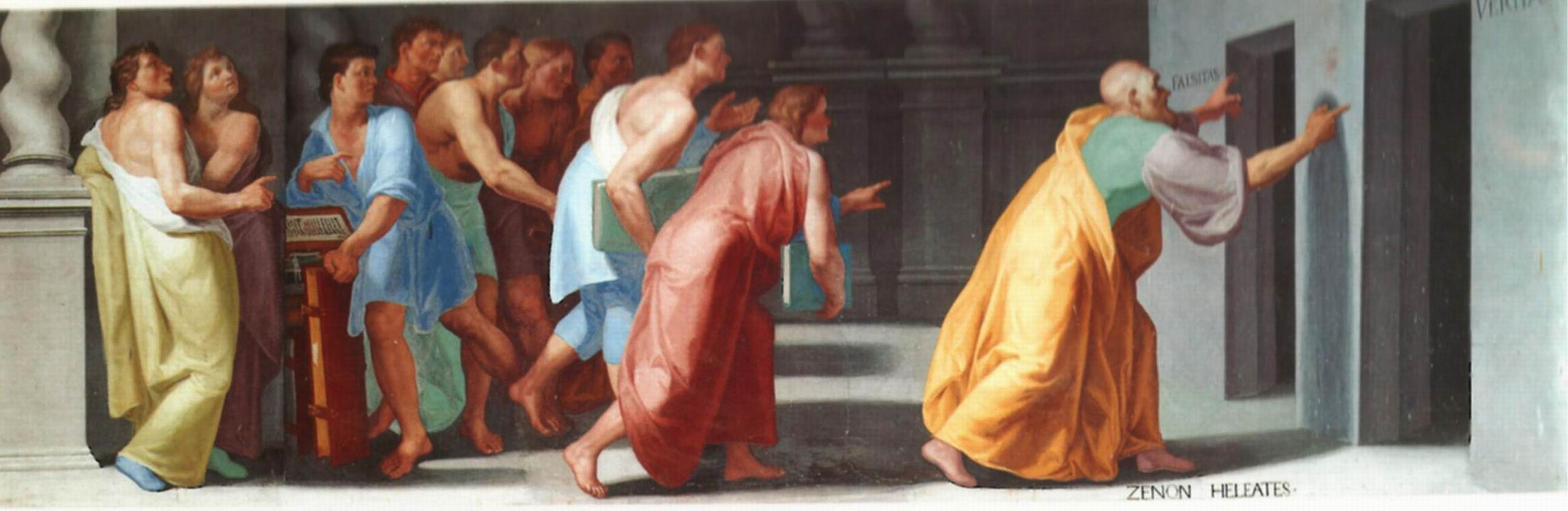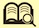Zeno of Elea (ca. 490–ca. 430) shows Youths the Doors to Truth and False.
Fresco in the Library of El Escorial, Madrid.
Date 1588–1595, by Bartolomeo Carducci or Pellegrino Tibaldi

• Calculus studies changing quantities and their rates of change. Zeno argued that change cannot occur. His arrow paradox: at any given instant, an arrow is at a fixed position; there is no motion.

The School of Athens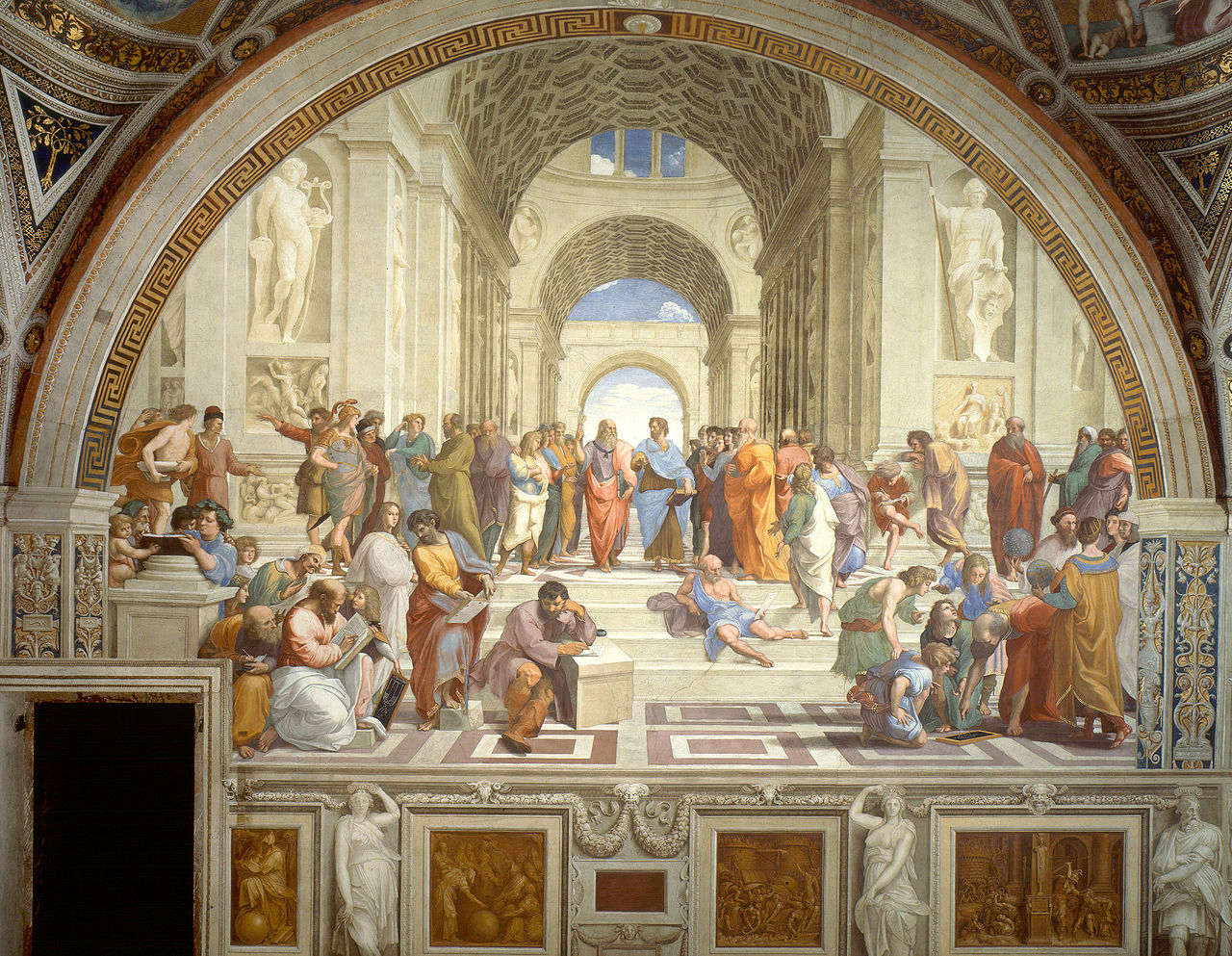Fresco in in the Apostolic Palace in the Vatican
Date 1509–1510, by Raphael
Includes figures for Pythagoras (ca. 570–ca. 490), Plato (427–347), Aristotle (384–322), Euclid (fl. ca. 295), Archimedes (287–212)

• Aristotle explained that time partakes of same character as space.

• Archimedes characterized uniform motion as that where a moving object traverses distances proportional to times. That means that the distance x traversed in a time interval of length t is proportional to the distance y traversed in a time interval of length s, that is, x/y = t/s. Roughly speaking, this means the number of times that the distance y goes into the distance x is the same as the number of the time s goes into the time t.

• Greek geometers and astronomers described nonuniform motion of planets by compounding uniform motion in circles. There was no general theory for nonuniform motions.

William of Ockham, from a stained glass window at a church in Surrey.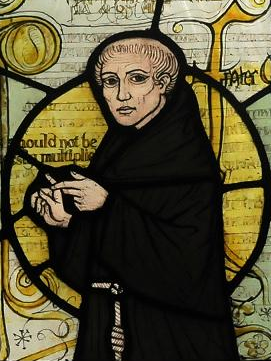William of Ockham (ca. 1285–ca. 1349)

• Ockham said that in order to have motion, all that’s necessary to be in different places at different times.

• By the 1300s scholars were comfortable with velocity, the rate of change of a changing quantity. Velocity, the ratio of distance to time, came to be accepted even though you can’t divide distance by time. Archimedes’ equation for uniform motion could be expressed as x/t = y/s, and that ratio called the constant velocity.

• Thomas Bradwardine, William Heytesbury, Richard Swineshead, and John Dumbleton— scholars at Merton College, Oxford—studied nonuniform motionin the early 1300s. They expressed and proved the Merton Mean Speed Theorem. It says that if an object is moving with a constant acceleration, then the distance it travels is the same distance it would travel if it were moving at a constant velocity, that velocity being the average of its initial and final velocity. (This happens to be the motion of a body in free fall, but there’s no indication that these Merton scholars knew that. That result was something Galileo (1564–1642) discovered much later.)

Miniature from Oresme’s Traité de l’espere, Bibliothèque Nationale, Paris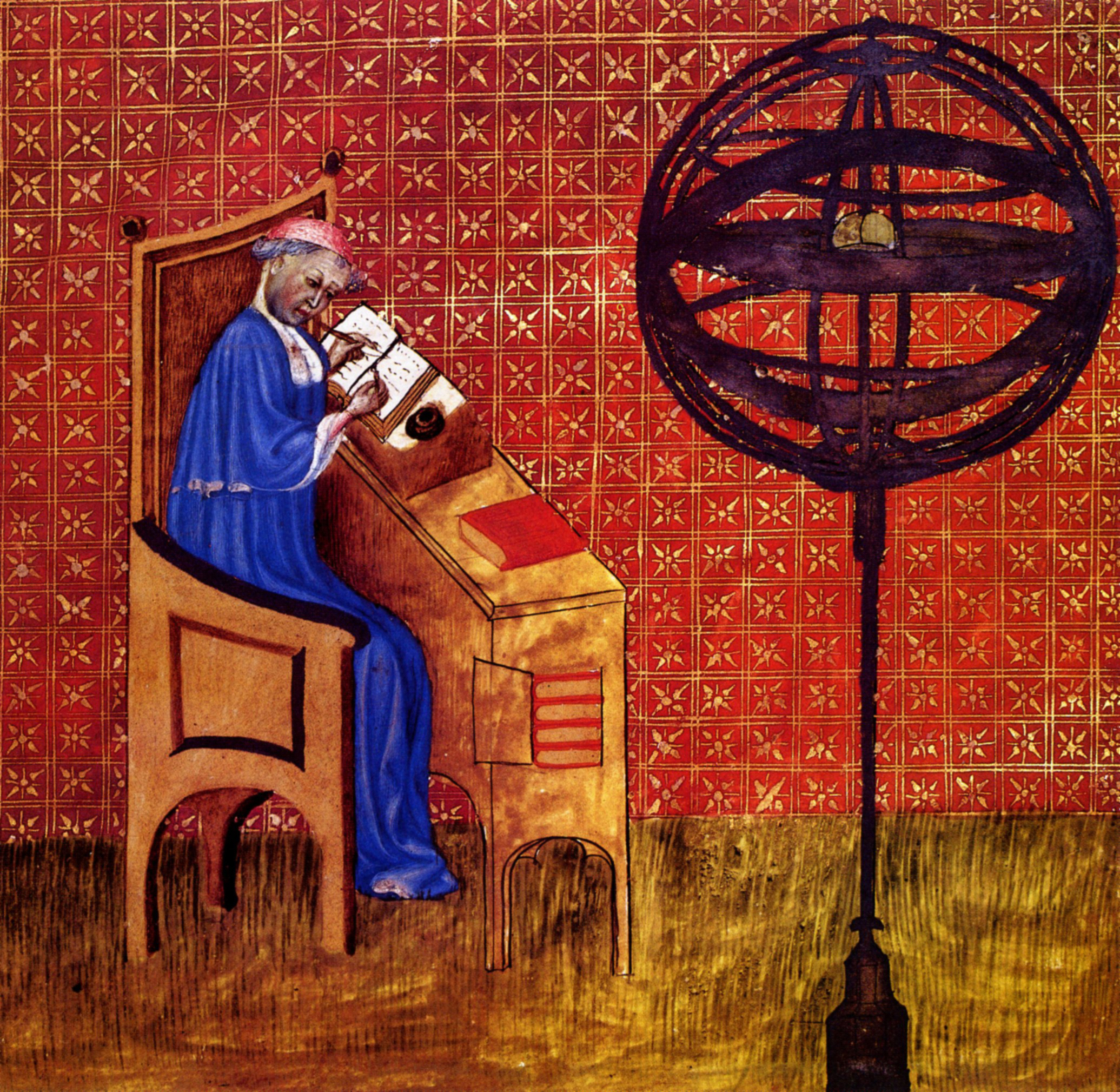Nicole Oresme (ca. 1320–1382)

Nicole Oresme was at the University of Paris and expanded the analytic study of changing quantities. He had a graphical interpretation very similar to the modern graph y = f(x) of a function in the (x,y)-plane, but analytic geometry and coordinates were yet to be developed by Fermat and Descartes in the 1600s.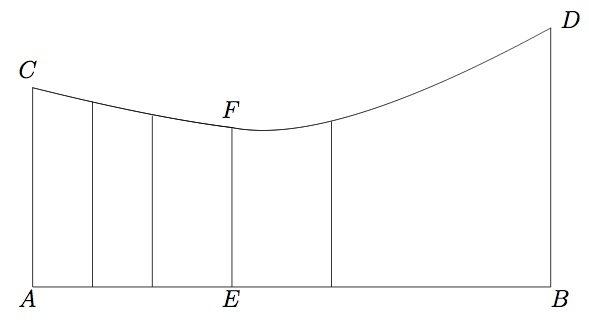He represented time as a line, much as Aristotle had done long before, so that instants in a time interval were represented by points on a horizontal line segment AB, which he called the longitude. Given a moving object, at each instant in time E that moving object has a velocity, and he represented that velocity by a vertical line segment EF proportional to the velocity; each vertical line segment he called a latitude. These latitudes together formed a plane region ABDC, which he called a form, bounded on the bottom by the original longitude AB, on the left by the initial latitude AC representing the initial velocity, on the right by the final latitude BD representing the final velocity, and on the top by the curve CFD which he called the summit curve. He then argued that the area of that form ABDC is proportional to the distance traveled.

This is now what is called the Fundamental Theorem of Calculus. It says that the area under the graph of the velocity equals the distance travelled. In modern terminology, that says the integral of the derivative equals the the total change in the function. In modern notation, that looks like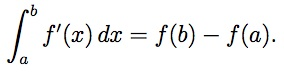Thus, the Fundamental Theorem of Calculus predates most of the rest of calculus by almost 300 years.

Dispute of Queen Cristina Vasa and René Descartes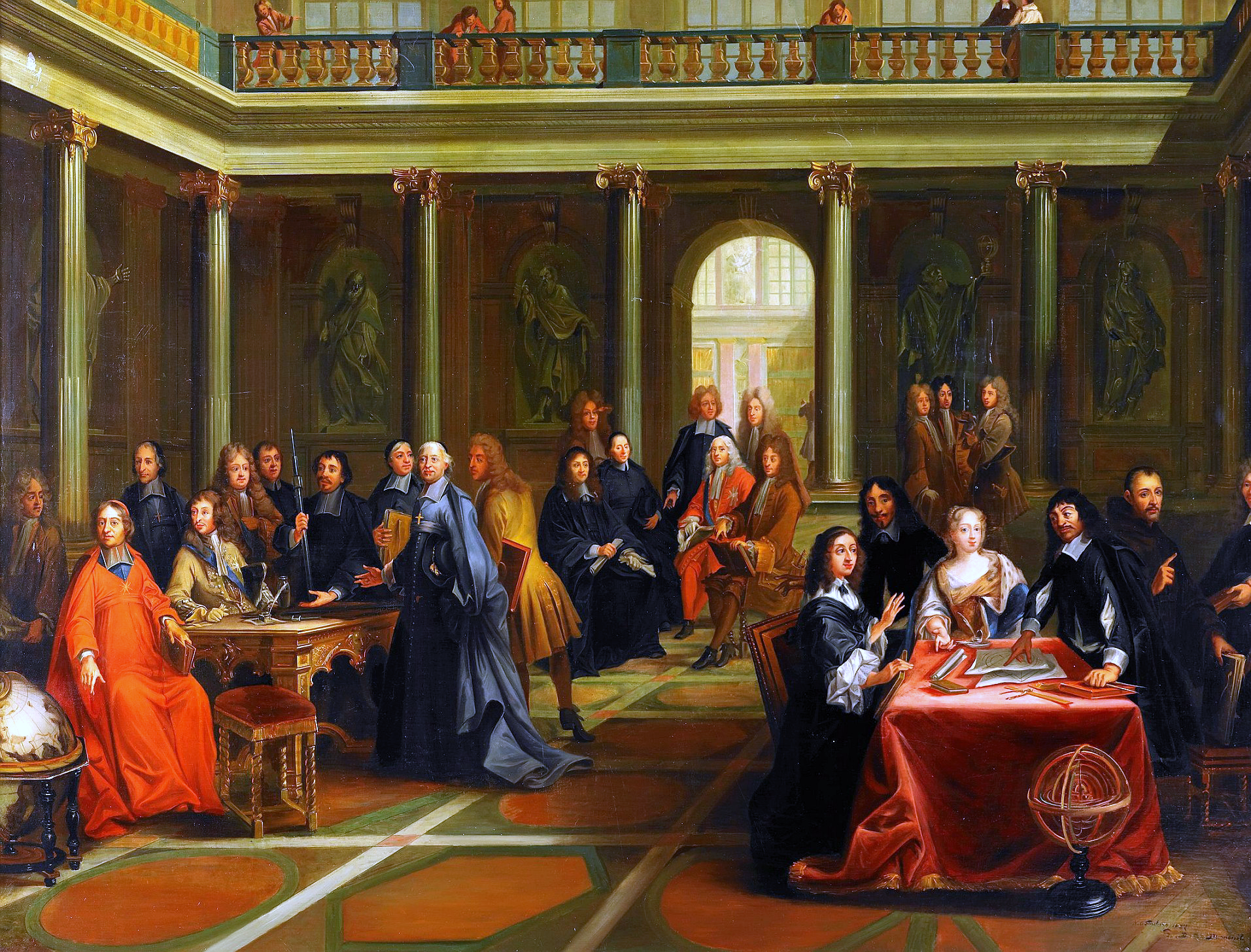Date 1884, by Nils Forsberg (1842–1934). Museo nacional de Versailles.
René Descartes (1596–1650)

• In the 16th century symbolic algebra was invented. Plus signs, minus signs, equal signs, square roots, etc., and variables x, y, and z.

• In the early 17th century Descartes and Fermat developed coordinate systems and analytic geometry. Equations in two variables correspond to curves in the plane. For example

 y2 = x3 – x + 1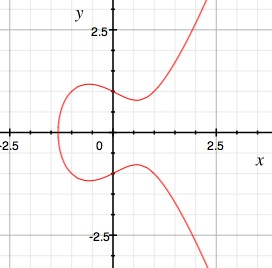Pierre de Fermat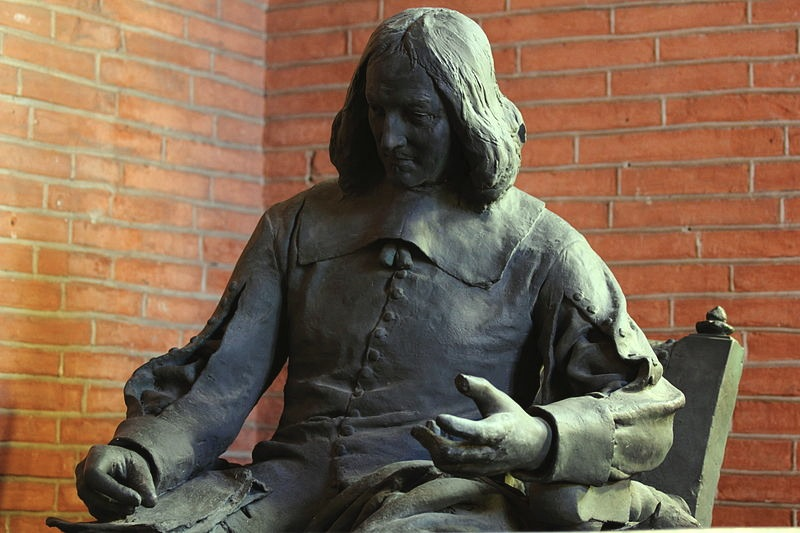Date 1886, by Alexandre Falguière (1831–1900). Musée des Augustins
Pierre de Fermat (1601–1665)

• Descartes and Fermat both immediately started studying properties of curves when they had analytic geometry. Descartes studied curvature, for example. Fermat developed many concepts of calculus

• Slope of a line. Change in y divided by change in x.

• A secant line is a line that cuts a curve. Slope of a secant line = average rate of change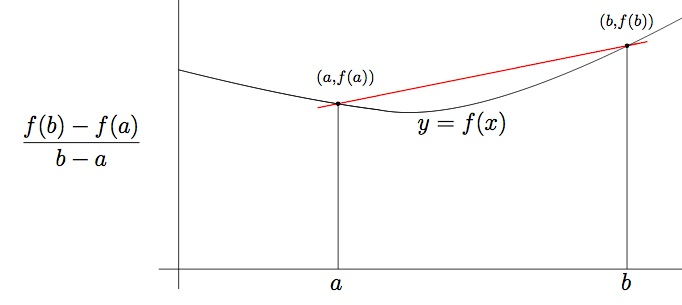• A tangent line is a line that touches a curve. Slope of a tangent line = instantaneous rate of change = derivative. Fermat imagined a tangent being a secant line that met the curve twice at the same point.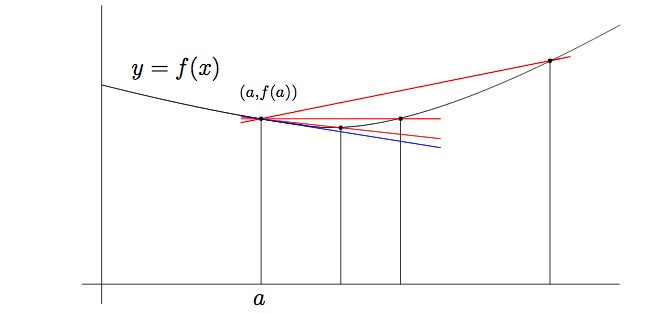• Fermat’s method: to find the slope of a tangent line, compute the slope of the secant line from a to b, simplify, then set b to a.

• Example. y = x2. Average rate of change is b2 – a2 divided by b – a. That simplifies to b + a. Set b to a to conclude that the slope of the tangent line is 2a.

• Fermat found derivatives of all polynomials and many other functions.

Portrait of Isaac Newton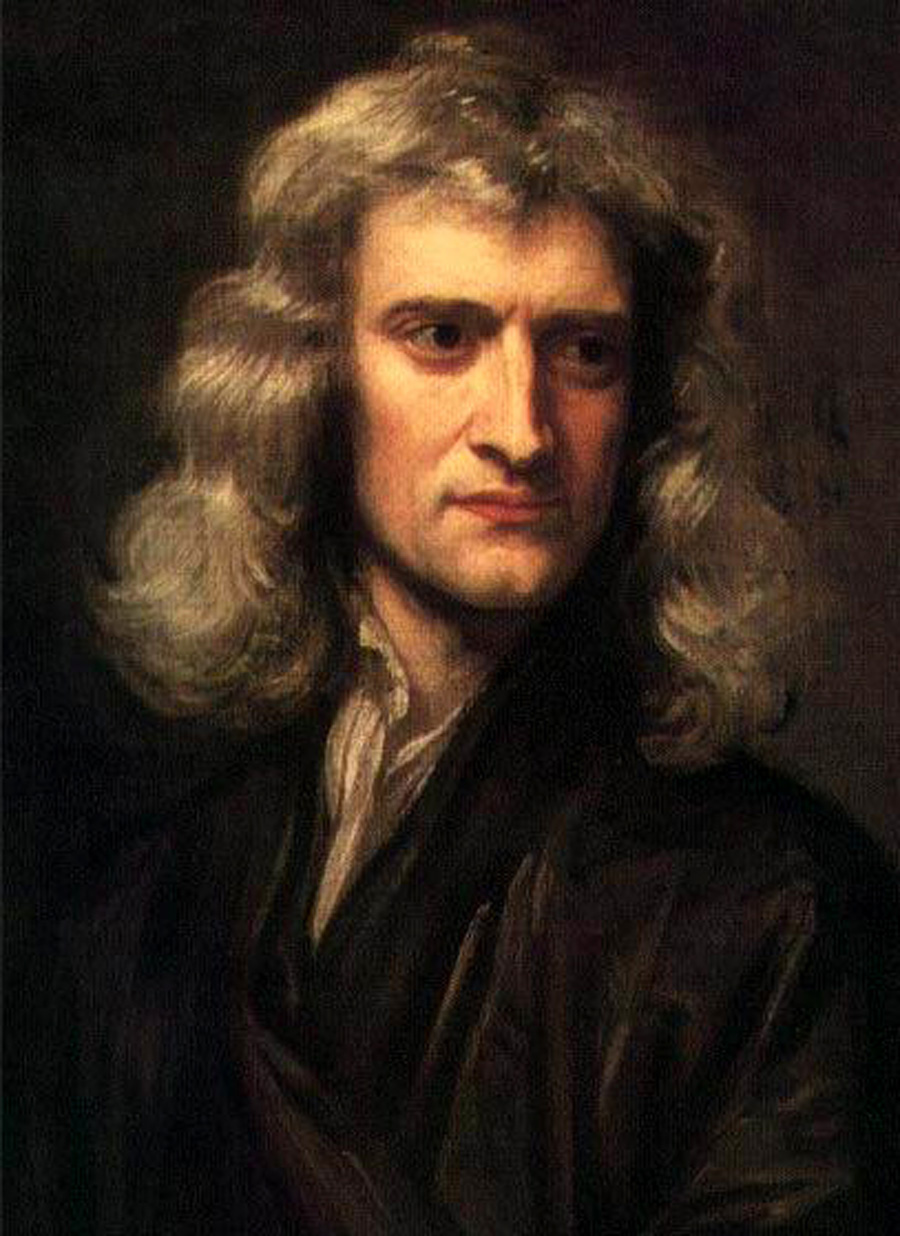Date 1689, by Sir Godfrey Kneller (1646–1723)
Isaac Newton (1642–1727)

 Newton and Leibniz are often called the founders of Calculus based on their work in the late 17th century But the Fundamental Theorem of Calculus, derivatives, and many other parts of calculus predated them They built their calculus on the mathematics of their predecessorsBritish two pound coins

A portrait of Gottfried Wilhelm von Leibniz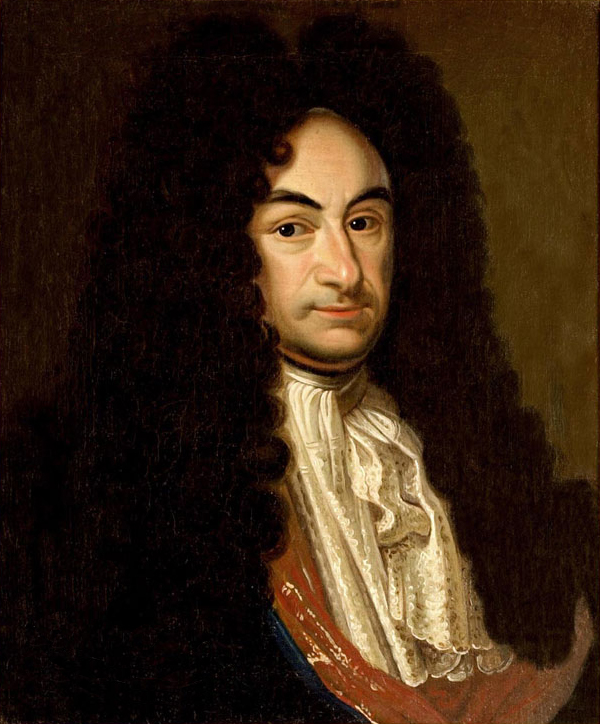17th century, artist unknown. Public Library of Hannover
Gottfried Wilhelm Leibniz (1646–1716)

• Newton’s approach to derivatives: follow Fermat but use limits as b approaches a rather than setting b to a

• Leibniz’ approach to derivatives: use infinitesimals, positive quantities that are smaller than any positive numbers